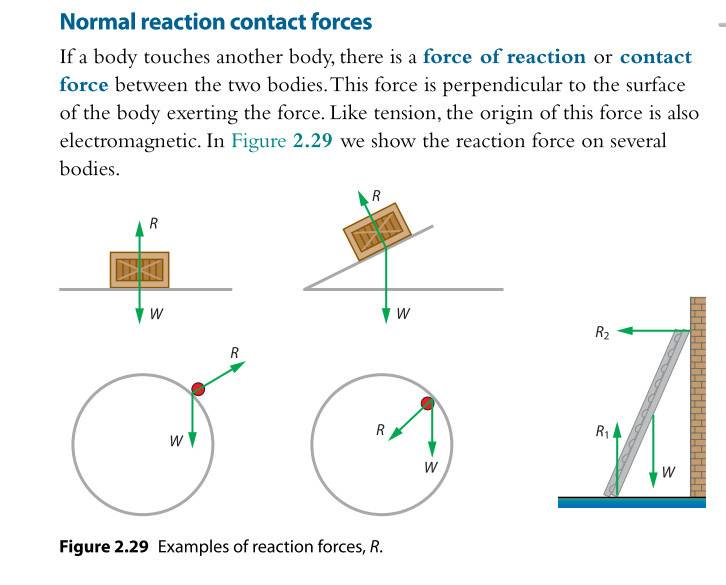# Where's the action force if the normal force is a reaction force?

I know that normal force is not a reaction force to the gravitational force;
- First, because the gravitational force is from Earth on the object (action force) thus the reaction of force is simply form the object on Earth according to Newton's third law.
- Also because in certain situations like when the object is on an inclined plane, the normal force doesn't even equal to the force of gravity on the object but only the perpendicular component of gravitational force.
- My question is: In some text books (attached is a screen of these), normal force is named "normal reaction force"; why is that and where's the action force if the normal force is a reaction force?#### Attachments

W?

Cheers

Chandra Prayaga
The word "Reaction" is unnecessary. The normal force is a force exerted by a surface on a body in contact with the surface. The body also (III law) exerts an equal force on the surface, in the opposite direction. These two are an action - reaction pair. Labeling one of them as "Action" and the other as "Reaction", is wrong.

A.T.
Labeling one of them as "Action" and the other as "Reaction", is wrong.
Or rather meaningless and confusing.

gmax137
W?

Cheers

Weight

jbriggs444
Homework Helper
Weight
In which case, at least 3 out of the 5 cases shown on the drawing are presented incorrectly. The weight vector is drawn originating from the centroid of the contact area rather than the center of mass of the object.

i.e. There is a difference between your weight (the downforce of gravity on your body) and the downward component of the contact force of your feet on the floor.

•gmax137
gmax137